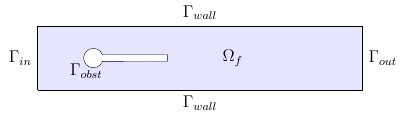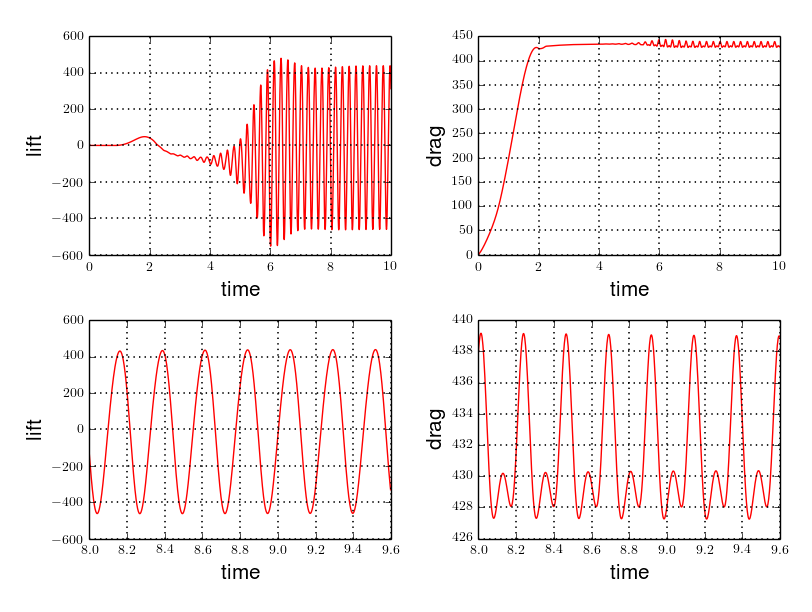# CFD Benchmarks

## 1. Introduction

We implement the benchmark proposed by [TurekHron], on the behavior of drag and lift forces of a flow around an object composed by a pole and a bar, see Figure Geometry of the Turek & Hron CFD Benchmark.

The software and the numerical results were initially obtained from [Chabannes].

 This benchmark is linked to the Turek-Hron CSM and Turek-Hron FSI benchmarks.

## 2. Problem Description

We consider a 2D model representative of a laminar incompressible flow around an obstacle. The flow domain, named $\Omega_f$, is contained into the rectangle $\lbrack 0,2.5 \rbrack \times \lbrack 0,0.41 \rbrack$. It is characterised, in particular, by its dynamic viscosity $\mu_f$ and by its density $\rho_f$. In this case, the fluid material we used is glycerine.Figure 1. Geometry of the Turek & Hron CFD Benchmark

In order to describe the flow, the incompressible Navier-Stokes model is chosen for this case, define by the conservation of momentum equation and the conservation of mass equation. At them, we add the material constitutive equation, that help us to define $\boldsymbol{\sigma}_f$

The goal of this benchmark is to study the behavior of lift forces $F_L$ and drag forces $F_D$, with three different fluid dynamics applied on the obstacle, i.e on $\Gamma_{obst}$, we made rigid by setting specific structure parameters. To simulate these cases, different mean inflow velocities, and thus different Reynolds numbers, will be used.

### 2.1. Boundary conditions

We set

• on $\Gamma_{in}$, an inflow Dirichlet condition : $\boldsymbol{u}_f=(v_{in},0)$

• on $\Gamma_{wall}$ and $\Gamma_{obst}$, a homogeneous Dirichlet condition : $\boldsymbol{u}_f=\boldsymbol{0}$

• on $\Gamma_{out}$, a Neumann condition : $\boldsymbol{\sigma}_f\boldsymbol{ n }_f=\boldsymbol{0}$

### 2.2. Initial conditions

We use a parabolic velocity profile, in order to describe the flow inlet by $\Gamma_{in}$, which can be express by

$v_{cst} = 1.5 \bar{U} \frac{4}{0.1681} y \left(0.41-y\right)$

where $\bar{U}$ is the mean inflow velocity.

However, we want to impose a progressive increase of this velocity profile. That’s why we define

v_{in} = \left\{ \begin{aligned} & v_{cst} \frac{1-\cos\left( \frac{\pi}{2} t \right) }{2} \quad & \text{ if } t < 2 \\ & v_{cst} \quad & \text{ otherwise } \end{aligned} \right.

With t the time.

Moreover, in this case, there is no source term, so $f_f\equiv 0$.

## 3. Inputs

The following table displays the various fixed and variables parameters of this test-case.

Table 1. Fixed and Variable Input Parameters
Name Description Nominal Value Units

$l$

elastic structure length

$0.35$

$m$

$h$

elastic structure height

$0.02$

$m$

$r$

$0.05$

$m$

$C$

cylinder center coordinates

$(0.2,0.2)$

$m$

$\nu_f$

kinematic viscosity

$1\times 10^{-3}$

$m^2/s$

$\mu_f$

dynamic viscosity

$1$

$kg/(m \times s)$

$\rho_f$

density

$1000$

$kg/m^3$

$f_f$

source term

0

$kg/(m^3 \times s)$

$\bar{U}$

characteristic inflow velocity

CFD1 CFD2 CFD3

$0.2$

$1$

$2$

$m/s$

## 4. Outputs

As defined above, the goal of this benchmark is to measure the drag and lift forces, $F_D$ and $F_L$, to control the fluid solver behavior. They can be obtain from

$(F_D,F_L)=\int_{\Gamma_{obst}}\boldsymbol{\sigma}_f \boldsymbol{ n }_f$

where $\boldsymbol{n}_f$ the outer unit normal vector from $\partial \Omega_f$.

## 5. Discretization

To realize these tests, we made the choice to used $P_N$-$P_{N-1}$ Taylor-Hood finite elements, described by [Chabannes], to discretize space. With the time discretization, we use BDF, for Backward Differentation Formulation, schemes at different orders $q$.

## 6. Running the case

The command line to run this case is

### 6.1. CFD1

``mpirun -np 4 feelpp_toolbox_fluid --case "github:{path:toolboxes/fluid/TurekHron}" --case.config-file cfd1.cfg``

### 6.2. CFD2

``mpirun -np 4 feelpp_toolbox_fluid --case "github:{path:toolboxes/fluid/TurekHron}" --case.config-file cfd2.cfg``

### 6.3. CFD3

``mpirun -np 4 feelpp_toolbox_fluid --case "github:{path:toolboxes/fluid/TurekHron}" --case.config-file cfd3.cfg``

## 7. Data files

The case data files are available in Github here

## 8. Results

Here are results from the different cases studied in this benchmark.

### 8.1. CFD1

Table 2. Results for CFD1
$\mathbf{N_{geo}}$ $\mathbf{N_{elt}}$ $\mathbf{N_{dof}}$ Drag Lift

Reference [TurekHron]

14.29

1.119

1

9874

45533 ($P_2/P_1$)

14.217

1.116

1

38094

173608 ($P_2/P_1$)

14.253

1.120

1

59586

270867 ($P_2/P_1$)

14.262

1.119

2

7026

78758 ($P_3/P_2$)

14.263

1.121

2

59650

660518 ($P_3/P_2$)

14.278

1.119

3

7026

146057 ($P_4/P_3$)

14.270

1.120

3

59650

1228831 ($P_4/P_3$)

14.280

1.119

All the files used for this case can be found in this rep [geo file, config file, json file]

### 8.2. CFD2

Table 3. Results for CFD2
$\mathbf{N_{geo}}$ $\mathbf{N_{elt}}$ $\mathbf{N_{dof}}$ Drag Lift

Reference [TurekHron]

136.7

10.53

1

7020

32510 ($P_2/P_1$)

135.33

10.364

1

38094

173608 ($P_2/P_1$)

136.39

10.537

1

59586

270867 ($P_2/P_1$)

136.49

10.531

2

7026

78758 ($P_3/P_2$)

136.67

10.548

2

59650

660518 ($P_3/P_2$)

136.66

10.532

3

7026

146057 ($P_4/P_3$)

136.65

10.539

3

59650

1228831 ($P_4/P_3$)

136.66

10.533

All the files used for this case can be found in this rep [geo file, config file, json file]

### 8.3. CFD3

As CFD3 is time-dependent ( from BDF use ), results will be expressed as

$mean ± amplitude [frequency]$

where

• mean is the average of the min and max values at the last period of oscillations.

$mean=\frac{1}{2}(max+min)$
• amplitude is the difference of the max and the min at the last oscillation.

$amplitude=\frac{1}{2}(max-min)$
• frequency can be obtain by Fourier analysis on periodic data and retrieve the lowest frequency or by the following formula, if we know the period time T.

$frequency=\frac{1}{T}$
Table 4. Results for CFD3
$\mathbf{\Delta t}$ $\mathbf{N_{geo}}$ $\mathbf{N_{elt}}$ $\mathbf{N_{dof}}$ $\mathbf{N_{bdf}}$ Drag Lift

0.005

Reference [TurekHron]

439.45 ± 5.6183[4.3956]

−11.893 ± 437.81[4.3956]

 0.01 1 8042 37514 ($P_2/P_1$) 2 437.47 ± 5.3750[4.3457] -9.786 ± 437.54[4.3457] 2 2334 26706 ($P_3/P_2$) 2 439.27 ± 5.1620[4.3457] -8.887 ± 429.06[4.3457] 2 7970 89790 ($P_2/P_2$) 2 439.56 ± 5.2335[4.3457] -11.719 ± 425.81[4.3457]
 0.005 1 3509 39843$(P_3/P_2)$ 2 438.24 ± 5.5375[4.3945] -11.024 ± 433.90[4.3945] 1 8042 90582 ($P_3/P_2$) 2 439.25 ± 5.6130[4.3945] -10.988 ± 437.70[4.3945] 2 2334 26706 ($P_3/P_2$) 2 439.49 ± 5.5985[4.3945] -10.534 ± 441.02[4.3945] 2 7970 89790 ($P_3/P_2$) 2 439.71 ± 5.6410[4.3945] -11.375 ± 438.37[4.3945] 3 3499 73440 ($P_4/P_3$) 3 439.93 ± 5.8072[4.3945] -14.511 ± 440.96[4.3945] 4 2314 78168 ($P_5/P_4$) 2 439.66 ± 5.6412[4.3945] -11.329 ± 438.93[4.3945]
 0.002 2 7942 89482 ($P_3/P_2)$ 2 439.81 ± 5.7370[4.3945] -13.730 ± 439.30[4.3945] 3 2340 49389 ($P_4/P_3$) 2 440.03 ± 5.7321[4.3945] -13.250 ± 439.64[4.3945] 3 2334 49266 ($P_4/P_3$) 3 440.06 ± 5.7773[4.3945] -14.092 ± 440.07[4.3945]

All the files used for this case can be found in this rep [geo file, config file, json file].Figure 2. Lift and drag forces

## 9. Geometrical Order

 Add a section on geometrical order.

## 10. Conclusion

The reference results of [TurekHron] have been obtained with a time step $\Delta t=0.05$. When we compare our results, with the same step and $\mathrm{BDF}_2$, we observe that they are in accordance with the reference results.

With a larger $\Delta t$, a discrepancy is observed, in particular for the drag force. It can also be seen at the same time step, with a higher order $\mathrm{BDF}_n$ ( e.g. $\mathrm{BDF}_3$ ). This suggests that the couple $\Delta t=0.05$ and $\mathrm{BDF}_2$ isn’t enough accurate.

## 11. Bibliography

References for this benchmark
• [TurekHron] S. Turek and J. Hron, Proposal for numerical benchmarking of fluid-structure interaction between an elastic object and laminar incompressible flow, Lecture Notes in Computational Science and Engineering, 2006.

• [Chabannes] Vincent Chabannes, Vers la simulation numérique des écoulements sanguins, Équations aux dérivées partielles [math.AP], Universitée de Grenoble, 2013.